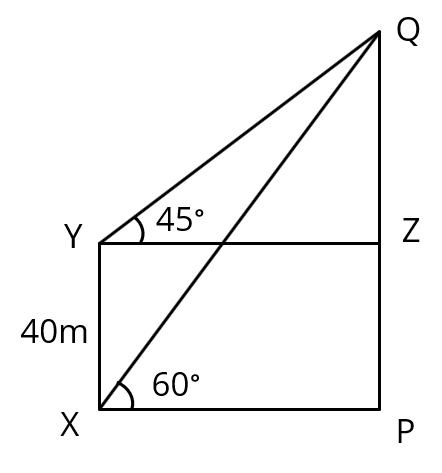# CBSE 2016 Maths Paper

10th Board Paper - Q26. Applications of Trigonometry

#### Question The angle of elevation of the top Q of a vertical tower PQ from a point x on the ground is 600. From a point y, 40 m vertically above x, the angle of elevation of the top Q of tower is 450. Find the height of the tower PQ and the distance PX. (Use $$sqrt3$ = 1.73). #### Video Explanation #### Explanatory AnswerPQ is the tower. XY = 40 m The angle of elevation of Q from X, ∠PXQ = 60o Draw line YZ parallel to XP The angle of elevation of Q from Y, ∠ZYQ = 45o What is to be found? PQ and PX Note: PX = ZY In right triangle QZY, tan45o = $\frac{QZ}{ZY}$ tan 45o = 1 $\frac{QZ}{ZY}$ = 1 or QZ = ZY Let us say ZY = QZ = d In right triangle QPX, tan60o = $\frac{QP}{PX}$ tan 60o = $\sqrt3$ QP = QZ + ZP = d + 40 PX = d $\sqrt3$ = $\frac{d + 40}{d}$ $\sqrt3$d = d + 40 $$$sqrt{3 - 1}$)d = 40 Or d = $\frac{40}{\sqrt{3-1}}$ = $\frac{40}{\sqrt{3-1}}$ × $\frac{\sqrt{3+1}}{\sqrt{3+1}}$ = $\frac{40{\sqrt{3+1}}}{3-1}$ = $\frac{40{\sqrt{3+1}}}{2}$ = 20$\sqrt{3+1}$ = 20$1.73 + 1)
= 20 × 2.73 = 54.6 m
So, d = PX = 54.6m

PQ = d + 40 = 54.6 + 40 = 94.6 m